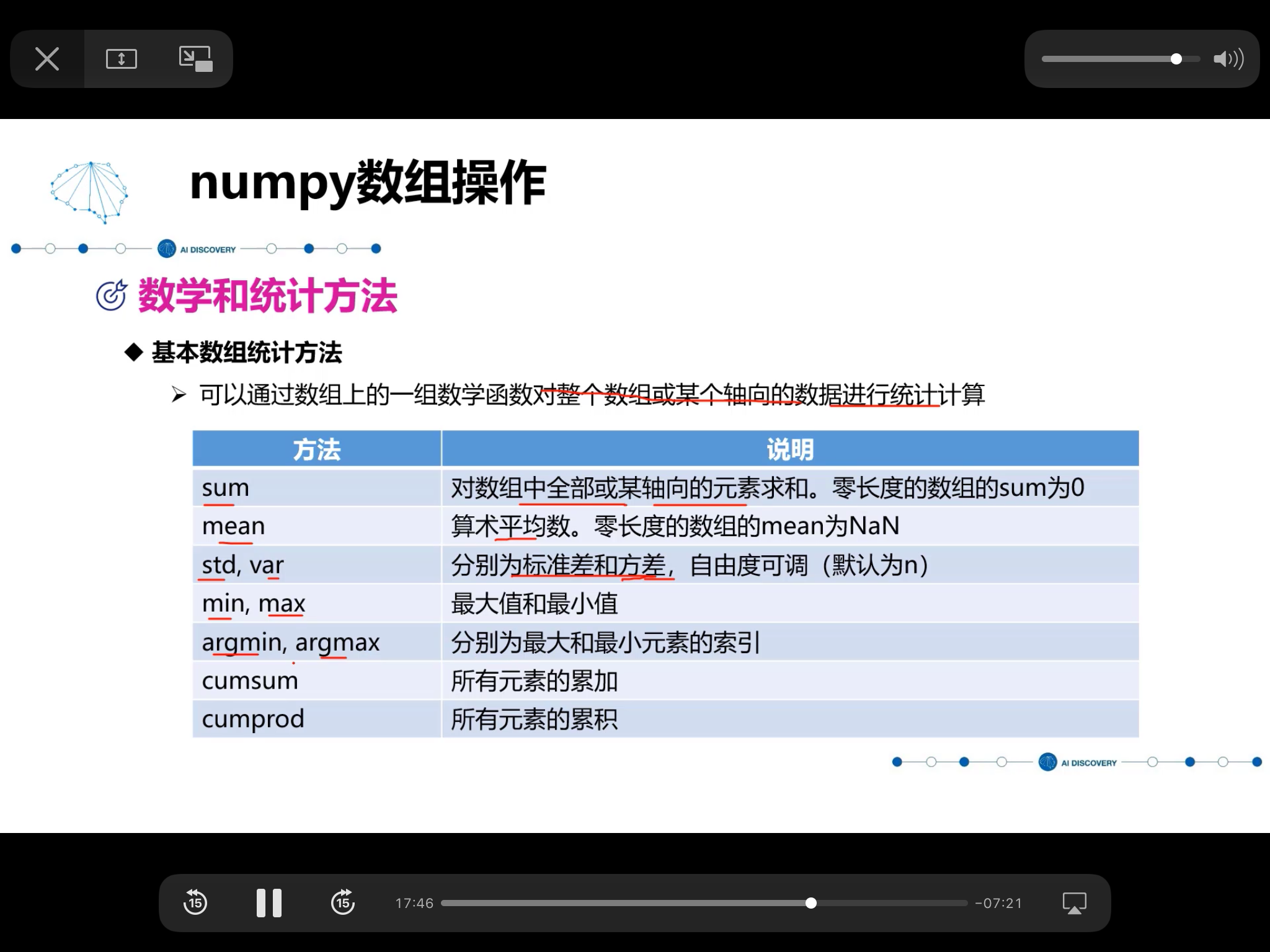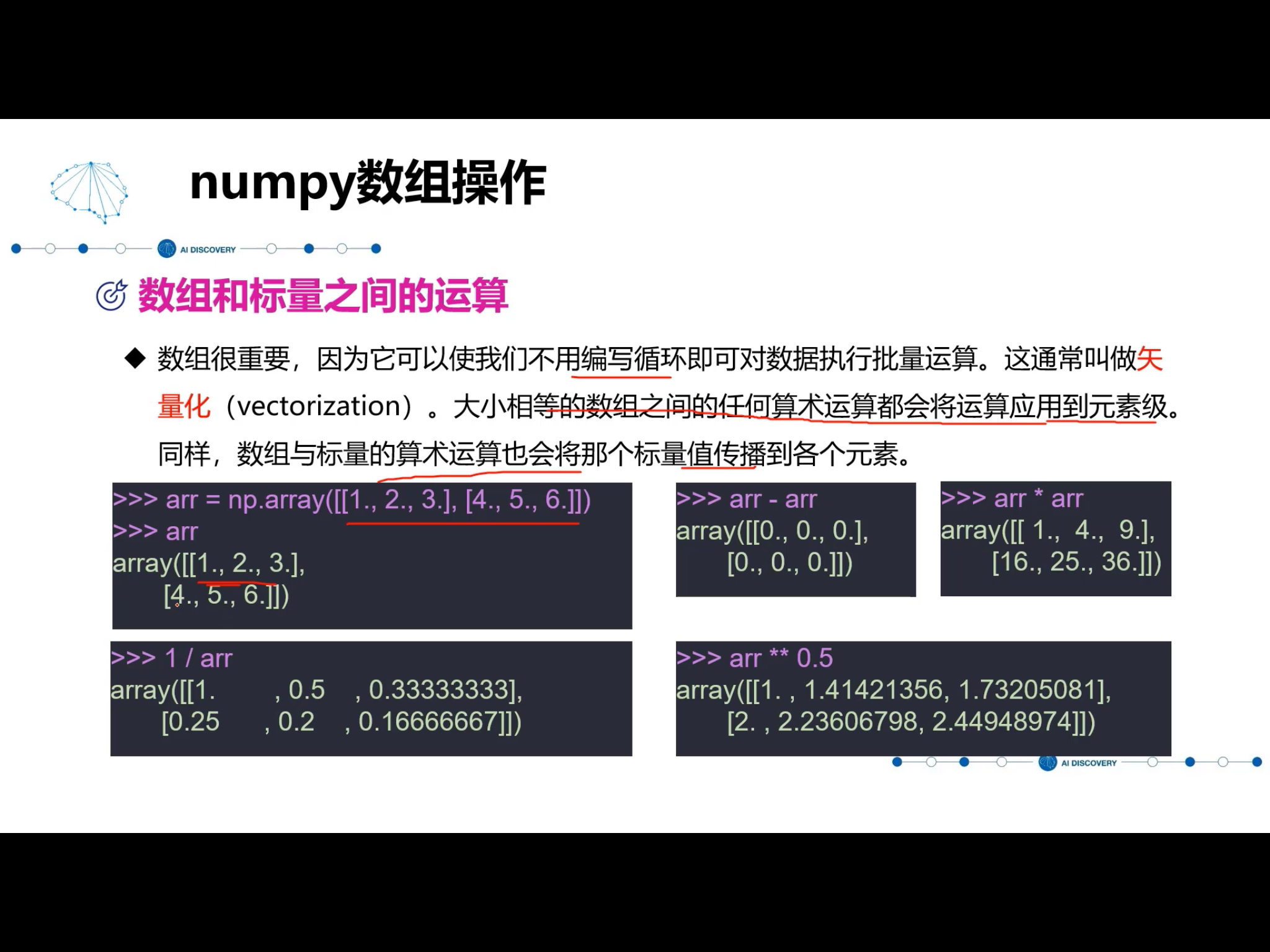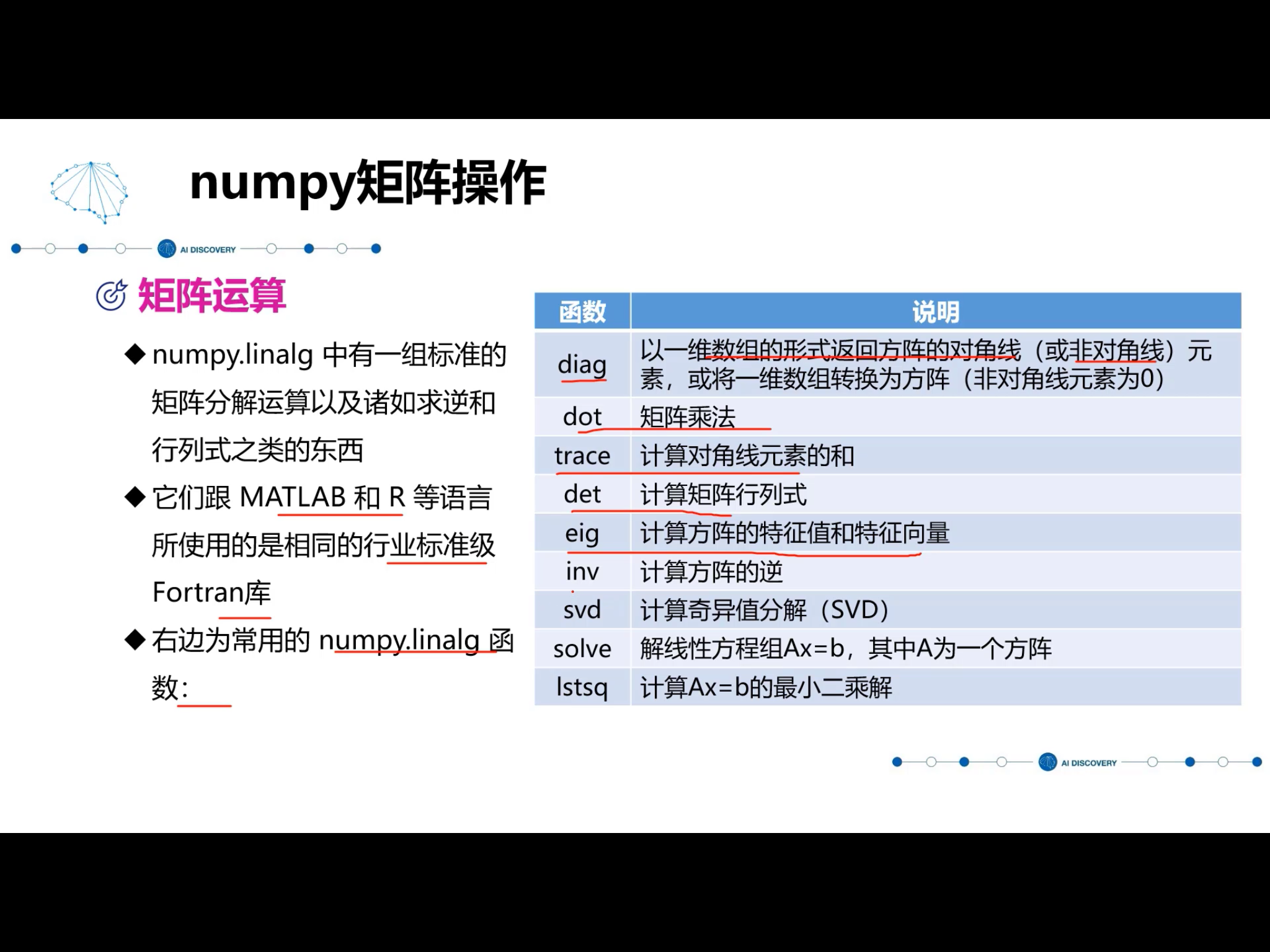# Python的数据处理

## numpy库的拓展

### numpy创建数组

import numpy as np
a = [1,2,3,4]
b = np.array(a) #将列表转换为数组



np.zeros()创建全零的数组
np.one（）

np.random.rand(10,10) 指定形状的（10行，10列）指定函数
—————.uniform(0,100)指定范围的一个数
—————.randint(0,100)创建指定范围的一个整数
—————.normal(1.75,0.1,(2,3))均值/标准差/维度的正态分布（2，3）的一个数组
randint(0,50,5)

randint(0,50,(3,5))
0~50的3行，5列的二维数组

### 数组标量之间的运算axis =0按照行来计算 =1 按照列进行计算## numpy的矩阵操作

numpy.matlib返回的是一个矩阵

import numpy as np
x=np.matrix([1,2,3],[4,5,6])
y=np.matrix([1,2],[3,4],[5,6])
print(x*y)©️2019 CSDN 皮肤主题: 游动-白 设计师: 上身试试